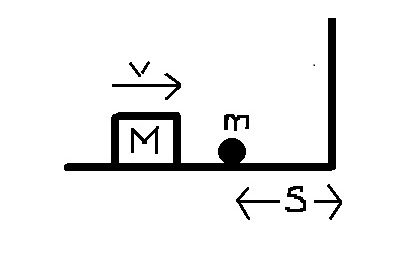# Collisions Galore!A block (with mass $M$ kg) moves towards a wall with velocity $v$ on a frictionless surface and collides elastically with a ball of mass $4$ kg. The ball moves towards the wall, collides elastically, and then continues to collide back and forth between the block and the wall.

The 10th time that the ball collides with the block, the block is left at rest and is at its closest approach to the wall. Find M.

Details and Assumptions

• The ball is initially at rest at a distance $S=411 \text{ m}$ from the wall.
• The block initially moves with $v=4 \text{ m/s}$.
• Kindly note that the $10^{th}$ collision refers to that between the block and the ball.
• This is part of Ordered Disorder.
×